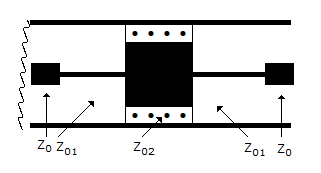# Electronics and Communication Engineering - Microwave Communication

### Exercise :: Microwave Communication - Section 3

1.

A cavity resonator is

 A. a hollow metallic enclosure B. a hollow enclosure having magnetic material as its walls C. a hollow enclosure having dielectric material as its walls D. either (b) or (c)

Explanation:

Hollow metallic enclosures exhibit resonance behaviour when excited by electromagnetic field.

These enclosures are called cavity resonators.

2.

If antenna diameter is increased four times, the maximum range is increased by a factor of

 A. 2 B. 2 C. 4 D. 0.2

Explanation:

Range of radar is proportional to antenna diameter.

3.

Assertion (A): Gunn diode is a transferred electron device.

Reason (R): A Gunn oscillator uses the phenomenon of transferred electron effect.

 A. Both A and R are correct and R is correct explanation of A B. Both A and R are correct but R is not correct explanation of A C. A is correct but R is wrong D. A is wrong but R is correct

Explanation:

A Gunn diode uses GaAs which has a negative differential mobility, i.e., a decrease in carrier velocity with increase in electric field.

This effects is called transferred electron effect. The impedance of a Gunn diode is tens of ohms.

A Gunn diode oscillator has a resonant cavity, an arrangement to couple Gunn diode to cavity, biasing arrangement for Gunn diode and arrangement to couple RF power to load.

4.

Atomic and molecular resonance is observed in many substances

 A. at microwave frequencies B. at low frequencies C. at frequencies used in AM broadcast D. at both (b) and (c)

Explanation:

Atomic and molecular distances are very small. Therefore resonance can occur only at microwave frequencies.

5.

The component in the given figure isA. attenuator B. T type low pass filter C. T type high pass filter D. phase shifter